Graphene Spin Susceptibility

R. B. Laughlin May 19, 2008

(Submitted as coursework for Physics 373, Stanford University, Spring 2008)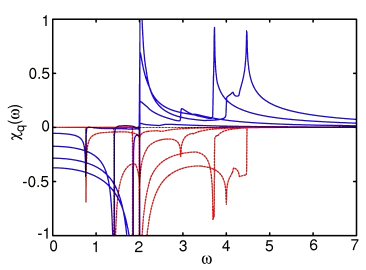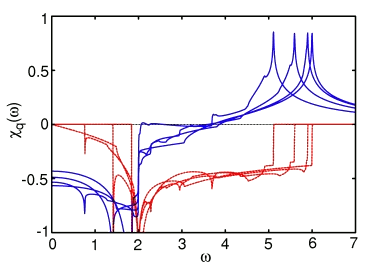Fig. 1: Real (blue) and imaginary (red) parts of the graphene spin susceptibility as a function of frequency for various values of q. Top: Four values of q from 0 to half a reciprocal lattice vector, or 2π/3 in the direction of a bond. Bottom: Four values of q from 2π/3 to 4π/3 in the same direction. The computer code that generated this graph is available here. I plotted using gnuplot. The plot file is shown here.

This report shows how the calculation for graphene's density of states described in my previous report can be modified to generate the spin susceptibility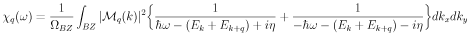It is functionally the same calculation except for inclusion of the matrix element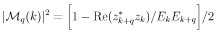where we have as before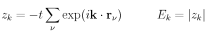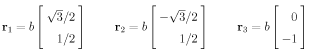As a practical matter we first compute the imaginary part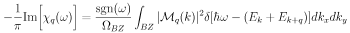where sgn denotes the sign, and then hilbert transform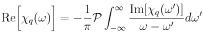The LaTeX source for the above equations is available here.